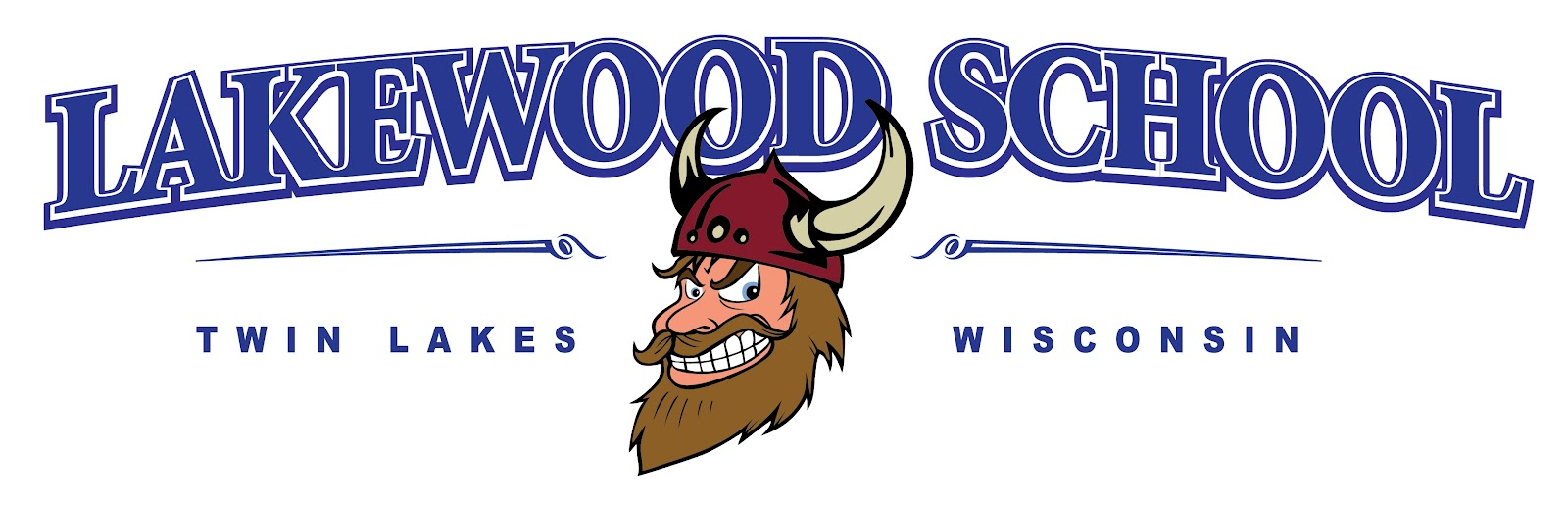Second Grade Curriculum Printable ELA English, Language Arts I can use the correct vowel sound when reading one syllable words. I know sounds for common vowel teams. I can decode two syllable words with long vowels. I can decode words with common prefixes and suffixes. I can identify homophones. I can read 2nd grade sight words. I can read on-level text for understanding. I can read on level text fluently. I can use context to help me read and self-correct if necessary. I can ask and answer questions to demonstrate understanding of a text. I can identify the main topic within a text. I can use text features to locate information. I can identify the main purpose of a text. I can compare and contrast points in two texts of the same topic. I can ask and answer questions about key details in a text. I can retell stories with key details and message. I can describe how characters in a story respond to major events and challenges. I can describe the structure of a story. I can acknowledge different points of view in a story. I can compare and contrast different versions of the same story. I can use linking words to connect my opinion and reasons, and write a concluding statement. I can introduce a topic, use facts and definitions and write a concluding statement. I can write about one long event or short events in a sequence using details to describe actions, thoughts and feelings. MATH Operations and Algebraic Thinking, Numbers and Operations in Base Ten, Measurement and Data, Geometry Use addition and subtraction within 100 to solve one- and two-step word problems involving situations of adding to, taking from, putting together, taking apart, and comparing, with unknowns in all positions Fluently add and subtract within 20 using mental strategies By end of Grade 2, know from memory all sums of two one-digit numbers Determine whether a group of objects (up to 20) has an odd or even number of members Write an equation to express an even number as a sum of two equal addends Understand that the three digits of a three-digit number represent amounts of hundreds, tens, and ones Count within 1000 Skip-count by 5s, 10s, and 100s Read and write numbers to 1000 using base-ten numerals, number names, and expanded form Compare two three-digit numbers based on meanings of the hundreds, tens, and ones digits, using >, =, and < symbols to record the results of comparisons Fluently add and subtract within 100 using strategies based on place value, properties of operations, and/or the relationship between addition and subtraction Add and subtract within 1000 Understand that in adding or subtracting three-digit numbers, one adds or subtracts hundreds and hundreds, tens and tens, ones and ones; and sometimes it is necessary to compose or decompose tens or hundreds Explain why addition and subtraction strategies work, using place value and the properties of operations Measure the length of an object by selecting and using appropriate tools such as rulers, yardsticks, meter sticks, and measuring tapes Estimate lengths using units of inches, feet, centimeters, and meters Tell and write time from analog and digital clocks to the nearest five minutes, using a.m. and p.m. Solve word problems involving dollar bills, quarters, dimes, nickels, and pennies, using \$ and ¢ symbols appropriately Generate measurement data by measuring lengths of several objects to the nearest whole unit, or by making repeated measurements of the same object Show the measurements by making a line plot, where the horizontal scale is marked off in whole-number units Draw a picture graph and a bar graph to represent a data set with up to four categories Solve simple put-together, take-apart, and compare problems using information presented in a bar graph Partition circles and rectangles into two, three, or four equal shares, describe the shares using the words halves, thirds, half of, a third of, etc., and describe the whole as two halves, three thirds, four fourths Recognize that equal shares of identical wholes need not have the same shape